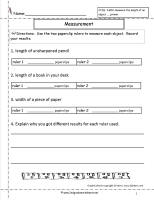﻿ CCSS 2.MD.2 Worksheets, Measuring and Estimating Lengths Worksheets

# Common Core State Standard 2.MD.2

## Measurement & Data

### Measure and estimate lengths in standard units.

Measure the length of an object twice, using length units of different lengths for the two measurements; describe how the two measurements relate to the size of the unit chosen.

###Measuring Worksheet

Common Core State Standards: 2.MD.2
Measurement & Data
Measure and estimate lengths in standard units.

Measure the length of an object twice, using length units of different lengths for the two measurements; describe how the two measurements relate to the size of the unit chosen.

Students measure using two different units.

###Measuring Worksheet

Common Core State Standards: 2.MD.2
Measurement & Data
Measure and estimate lengths in standard units.

Measure the length of an object twice, using length units of different lengths for the two measurements; describe how the two measurements relate to the size of the unit chosen.

Students measure using two different units.